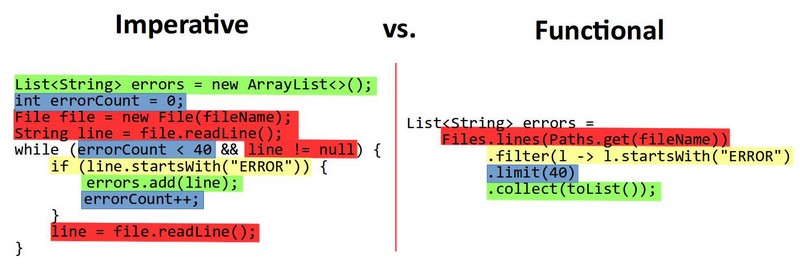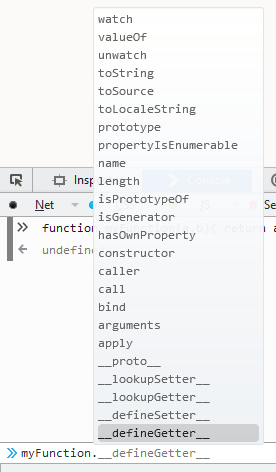# Javascript - Arrow Function Expression

An arrow function expression has a shorter syntax than a function expression and does not bind its own:

• this,
• arguments,
• super,
• or new.target.

These function expressions are best suited for non-method functions.

As an arrow function does not:

• bind its own this, it doesn't create then its own this context, this has its original meaning from the enclosing context (even in non strict).
• bind an arguments object. Arguments are simply a reference to the same name in the enclosing scope.
• bind to new.target. It cannot be used as constructors and will throw an error when used with new.

## Syntax

### Javascript

``````(param1 = defaultValue1, param2, …, paramN = defaultValueN) => { statements }
(param1 = defaultValue1, param2, …, paramN = defaultValueN) => expression  // equivalent to { return expression; }```
```

where:

• parentheses are optional with one parameter
• parentheses are mandatory without parameter and for several parameters
• defaultValue1…N are default parameter

The following syntax is also supported:

### Typescript

``````const myFunction = (param1: Type1, param2: Type2): ReturnType => {
// function body
}```
```

## Example

### Circle Area

``````var area = (r) => Math.PI * r ** 2;
var rayon = 3;
console.log(`The area of a circle with the rayon \${rayon} is \${area(rayon)}`);```
```

### Destructuring Assignment

````var f = ([a, b] = [1, 2], {x: c} = {x: a + b}) => a + b + c;`
```

where:

• in [a, b] = [1, 2]:
• a get the value 1
• b get the value 2
• in {x: c} = {x: a + b}
• c get the value a + b thus 3
• then a + b + c equal:
````console.log(f());  // 6`
```

## Documentation / Reference

Recommended Pages
CommonJs (NodeJs) - Require

The import functionality implementation of commonjs (and then node) is done via the require function require basically: reads a Javascript file (generally a CommonJs module but it may be any javascript...
ES - ES6 Harmony (ES2015)

ECMAScript 6 (also known as ES2015). The first ECMAScript Harmony specification, it is also known as ES6 Harmony class declarations (class Foo...Functional Programming - Map

A page the map functional programming function The map method creates a new collection with the results of calling a provided function on every element in the collection. The map operation produces...Javascript - Functions

Functions functionalities in Javascript. A function in Javascript is a variable that’s given a reference to the function being declared. The function itself is a value (as an integer or an array) that...
Javascript - Map function (Functional programming)

The functional programming function map in a Javascript context is only an array method as all functional programming javascript method. map With an Arrow function as argument Letters that we...